# How to Automate an Excel Sheet in Python?

Data storage, analysis, and presentation are frequently done using Excel. The popular programming language Python, on the other hand, is renowned for its ease of use, adaptability, and versatility. For use with Excel spreadsheets and for various task automation, Python offers a number of libraries. We can easily make use of Python for automating Excel spreadsheets. For doing the same, we will be covering various approaches in this article:

### Approaches

• Using the openpyxl library

• Using the pandas library

• Using the xlwings library

## Method 1: Using the openpyxl library

We can use the Python Openpyxl package for interacting with Excel files. This library allows us to read, write, and edit Excel files.

We can install openpyxl module using the following command:

pip install openpyxl

Suppose there is an excel file with the following contents −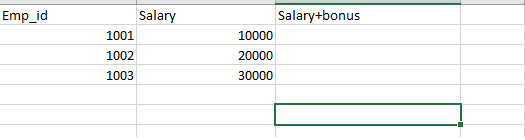Now, we want to automatically add the data in the new field for storing bonus+ salary of a particular employee. For this, we will write some code based on the below algorithm.

### Algorithm

• Import the necessary modules

• Load the Excel workbook 'python_worksheet.xlsx' using the openpyxl.load_workbook() function and assign it to the variable 'wb'.

• Select the first sheet of the workbook using wb['Sheet1'] and assign it to the variable named 'sheet'.

• Loop through each row in the sheet starting from the second row:

• Access the cell in the second column of the current row using sheet.cell(row, 2) and assign it to the variable 'cell'.

• Access the cell in the third column of the current row using sheet.cell(row, 3) and assign it to the variable 'salary_bonus_cell'.

• Add 500 to the value of 'cell' using int(cell.value) + 500 and assign the result to the value of 'salary_bonus_cell' using salary_bonus_cell.value = int(cell.value) + 500.

• Save the modified workbook with a new filename 'python-spreadsheet2.xlsx' using wb.save('python-spreadsheet2.xlsx').

Step 1 − Import necessary modules

import openpyxl as xl
from openpyxl.chart import BarChart, Reference


Step 2 − Load the workbook and select the worksheet

wb = xl.load_workbook('python_worksheet.xlsx')
sheet = wb['Sheet1']


Step 3 − Loop through each row starting from the second row and perform the calculations as per need.

for row in range(2, sheet.max_row + 1):
cell = sheet.cell(row, 2)
salary_bonus_cell = sheet.cell(row, 3)
salary_bonus_cell.value = int(cell.value) + 500


Step 4 − Save the modified workbook with a new filename

wb.save('python-spreadsheet2.xlsx')


### Example

# Importing the necessary modules
import openpyxl as xl

sheet = wb['Sheet1']

# Looping through each row of the sheet starting from row 2
for row in range(2, sheet.max_row + 1):
# Accessing the cell in the second column of the current row
cell = sheet.cell(row, 2)

# Accessing the cell in the third column of the current row
salary_bonus_cell = sheet.cell(row, 3)

# Adding 500 to the value in the cell from column 2 and updating the value in column 3
salary_bonus_cell.value = int(cell.value) + 500

# Saving the modified workbook with a new filename


The openpyxl method involves reading straight from the Excel files and writing to Excel files using the openpyxl module. We got to know how to open an Excel file, edit its data and make new column values. While this method can be used to work with single cells as well as ranges of cells, the code can be too lengthy and less effective for bigger datasets than the second method due to some limitations of this library.

### Output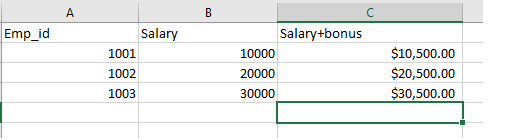## Method 2: Using the pandas library

Pandas is a Python package mostly utilized for data analysis. It also offers the ability to read and write Excel files, though. An illustration of how to automate an Excel sheet using pandas is given below:

We can install pandas in python using the following command:

pip install pandas

Suppose there is an excel file with the following contents: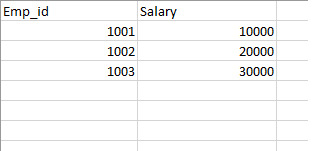Now, we want to do the same operation as described in the last example, we will be following the below algorithm:

### Algorithm

• Import the pandas library as pd.

• Use the pd.read_excel() method to read the data from an Excel file.

• Specify the sheet name “Sheet1” to read from the Excel file.

• Manipulate the data as needed using the various methods available in pandas.

• Create a new column in the DataFrame named "Bonus" that adds 1000 to the "Salary" column.

• Use the to_excel() method to write the updated data back to a new Excel file and also Specify index=False to prevent pandas from writing the DataFrame's index to the output file.

Step 1 − Import the pandas library using the statement "import pandas as pd".

import pandas as pd


Step 2 − Read the input Excel file "python_worksheet.xlsx" using the pd.read_excel() method and store it in a variable called "data".

data = pd.read_excel('python_worksheet.xlsx')


Step 3 − Specify the sheet name as "Sheet1" in the pd.read_excel() method to read data from the Sheet1 worksheet of the input Excel file.

data = pd.read_excel('python_worksheet.xlsx', sheet_name='Sheet1')


Step 4 − Create a new column in the "data" DataFrame called "Bonus" by adding 1000 to the existing "Salary" column. You can do this using the "+" operator and saving the result in a new column.

data['Bonus'] = data['Salary'] + 1000


Step 5 − Write the updated data back to a new Excel file called "python-spreadsheet2.xlsx" using the to_excel() method. Pass the DataFrame "data" as the first argument and specify index=False to prevent the DataFrame's index from being written to the output file.

data.to_excel('python-spreadsheet2.xlsx', index=False)


Step 6 − Run the code and check the output Excel file "python-spreadsheet2.xlsx" to verify that the "Bonus" column has been added to the original data.

### Example

# Import the pandas library
import pandas as pd

# Read the input Excel file "python_worksheet.xlsx" into a DataFrame called "data"
# and specify the sheet name as "Sheet1"

# Create a new column in the "data" DataFrame called "Bonus" by adding 1000 to the existing "Salary" column
data['Bonus'] = data['Salary'] + 1000

# Write the updated data back to a new Excel file called "python-spreadsheet2.xlsx"
# Pass the DataFrame "data" as the first argument and specify index=False to prevent the DataFrame's index from being written to the output file


The pandas method involves reading and writing data in Excel files by using DataFrames. In our example, we showed how to take data from an Excel file, automate adding a new column, and then putting all the new data to another Excel file. As pandas provides a wide collection of capabilities for manipulating, cleaning, and analysis of data, this method is helpful even when working with huge datasets.

### Output

In this example, we have automated the excel sheet for populating the Bonus column using python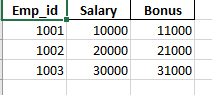## Method 3: Using the xlwings library

Xlwings is a python library that gives the users a way to communicate and automate processes with Microsoft Excel from Python. It enables users to automate Excel activities like creating and editing Excel charts and tables using Python, as well as write Excel formulas and functions.

Also, we can do calculations and analysis on Excel data using Python functions and libraries, and then write the findings back into Excel.

We can install xlwings in python using the following command:

pip install xlwings

Suppose there is an excel file with the following contents −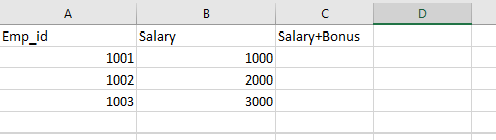Now, we want to do the same operation as described in the last example, we will be following the below algorithm:

### Algorithm

• Import the xlwings module.

• Load the Excel file into an xlwings Workbook object.

• Select the Worksheet and determine the last row of the 'Salary' column.

• Add 500 to each value in the 'Salary' column to create a new 'Bonus' column.

• Write the result to the corresponding 'Bonus' cell.

• Save the modified workbook with a new filename.

• Close the workbook

Step 1 − Import the xlwings module.

import xlwings as xw


Step 2 − Load the Excel file into an xlwings Workbook object

wb = xw.Book('python_worksheet.xlsx')


Step 3 − Select the Worksheet and add 500 to the 'Salary' column to create a new 'Bonus' column

sheet = wb.sheets['Sheet1']
last_row = sheet.range('B' + str(sheet.cells.last_cell.row)).end('up').row
sheet.range('C2:C' + str(last_row)).value = [[cell.value + 500] for cell in sheet.range('B2:B' + str(last_row))]


Step 4 − Save the modified workbook with a new filename

wb.save('python-spreadsheet2.xlsx')


Step 5 − Close the workbook

wb.close()


### Example

# Import the xlwings module
import xlwings as xw

# Load the Excel file into an xlwings Workbook object
wb = xw.Book('python_worksheet.xlsx')

# Select the Worksheet and add 500 to the 'Salary' column to create a new 'Bonus' column
sheet = wb.sheets['Sheet1']
last_row = sheet.range('B' + str(sheet.cells.last_cell.row)).end('up').row
sheet.range('C2:C' + str(last_row)).value = [[cell.value + 500] for cell in sheet.range('B2:B' + str(last_row))]

# Save the modified workbook with a new filename

# Close the workbook
wb.close()


This approach involves using the xlwings library, which allows you to automate and manipulate Excel through Python code. Due to this library’s speciality of working with Excel files, we can make use of this approach easily for automating our large excel tasks.

### Output

We have successfully added a column for Salary+Bonus in our excel sheet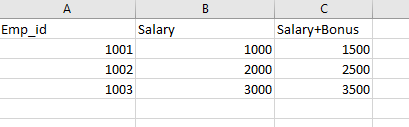## Conclusion

Automating Excel sheets using Python can be a useful tool for handling huge datasets, streamlining redundant and repetitive tasks, and producing larger reports. We've covered three popular methods in this article for automating Excel sheets in Python. Ultimately, the method you choose will rely on the requirements of the tasks as well as the volume and complexity of the data. Automating Excel sheets in Python can help you save time, cut down on manual errors, and boost productivity if you use the correct strategy and equipment.

Updated on: 29-May-2023

732 Views